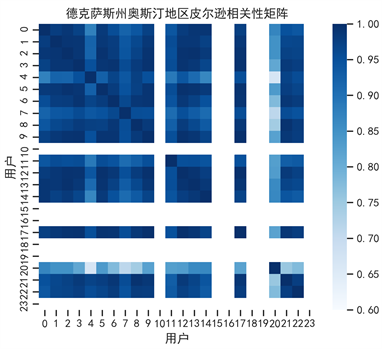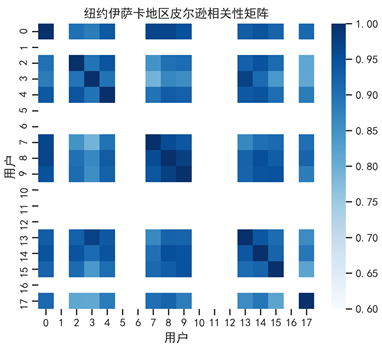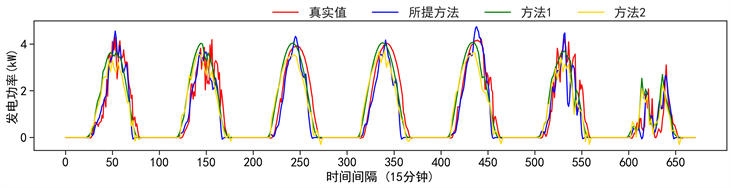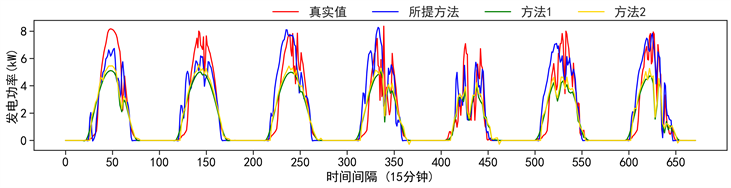#### 期刊菜单

Maximal Information Coefficient Based Residential Photovoltaic Power Generation Disaggregation
DOI: 10.12677/SG.2022.122007, PDF, HTML, XML, 下载: 163  浏览: 235

Abstract: Due to policy support, low cost and easy applicability, distribution photovoltaic systems (DPVSs) are increasingly popular among residential community. However, small-scale DPVSs of less than 10 kWp are always installed behind the meter (BTM), without metering the photovoltaic (PV) power generation separately, which results in the invisible of the PV power generation. Only access of net load data can result in non-optimal distribution network control and optimization, leading to a series of energy management problems. In order to solve the aforementioned problems, this paper proposes a BTM net load disaggregation method focusing on small-scale DPVSs, with only net load data of residential users in a community, without relying on weather data and models assumption. Considering that community users’ DPVSs usually exhibit approximate output characteristics, neighboring net load is used to extract PV power generation information as mutual proxies. After obtaining approximate PV proxy data by subtracting composite power of inter-users, Maximal Information Coefficient (MIC) is performed to obtain final PV power generation disaggregation results. Testing results show that the proposed method achieves considerable disaggregation accuracy in the absence of weather data.

1. 引言

2. BTM光伏发电量分解算法介绍

2.1. 使用社区用户总净负荷筛选PV代理站点

${C}_{i,t}={D}_{i,t}-{P}_{i,t},i\in n$ (1)

${{C}^{\prime }}_{i,t}=\frac{{C}_{i,t}}{\frac{1}{m}\underset{{t}_{1}=1}{\overset{m}{\sum }}{C}_{i,{t}_{1}}},i\in n,{t}_{1}\in {T}_{night}$ (2)

${{C}^{\prime }}_{i,t}-{{C}^{\prime }}_{j,t}=\left({{D}^{\prime }}_{i,t}-{{D}^{\prime }}_{j,t}\right)-\left({P}_{i,t}-{{P}^{\prime }}_{j,t}\right);i\in n,j\in n,i (3)

$\Delta {{C}^{\prime }}_{ij,t}=\Delta {{D}^{\prime }}_{ij,t}-\Delta {{P}^{\prime }}_{ij,t}$ (4)

$\Delta {{C}^{\prime }}_{ij,t}\approx -\Delta {{P}^{\prime }}_{ij,t}$ (5)

${I}_{i,t}={I}_{j,t}$ (6)

$P=\epsilon \cdot \alpha \cdot I$ (7)

$\frac{{{P}^{\prime }}_{i,t}}{{\alpha }_{i}}=\frac{{{P}^{\prime }}_{j,t}}{{\alpha }_{j}}$ (8)

${{P}^{\prime }}_{i,t}={\beta }_{ij}\Delta {{P}^{\prime }}_{ij,t}$ (9)

${\beta }_{ij}={\alpha }_{i}/\left({\alpha }_{i}-{\alpha }_{j}\right)$, ${\alpha }_{i}\ne {\alpha }_{j}$ (10)

$\frac{{{P}^{\prime }}_{i,t}}{{\alpha }_{i}}=\frac{{\beta }_{ij}}{{\alpha }_{i}}\Delta {{P}^{\prime }}_{ij,t}$ (11)

$\Delta {{P}^{\prime }}_{ij,t}$ 可以看作，装机容量为 $\frac{{\alpha }_{i}}{{\beta }_{ij}}$ 的PV设备在t时刻的PV发电量，也即是本文所提的PV代理站

${R}_{1}=\mathrm{arg}\underset{i,j}{\mathrm{max}}\text{MIC}\left({{C}^{\prime }}_{n,t},\Delta {{C}^{\prime }}_{ij,t}\right)$ (12)

2.2. 使用最大信息系数估计用户PV发电量

${{P}^{\prime }}_{i,t}={\beta }_{i}\Delta {P}_{t};i\in n$ (13)

${R}_{2}=\mathrm{arg}\underset{{\beta }_{i}}{\mathrm{min}}\text{MIC}\left({{C}^{\prime }}_{i,t}+{\beta }_{i}\Delta {P}_{t},\Delta {P}_{t}\right)$ (14)

${\stackrel{^}{P}}_{i,t}={{P}^{\prime }}_{i,t}\cdot \frac{1}{m}\underset{{t}_{1}=1}{\overset{m}{\sum }}{C}_{i,{t}_{1}}$ (15)

3. 算例分析

3.1. 数据集及评价指标

$\text{RMSE}=\sqrt{\frac{1}{T}\underset{t=1}{\overset{T}{\sum }}{\left({\stackrel{^}{P}}_{i,t}-{P}_{i,t}\right)}^{2}}$ (16)

$\text{CV}=\frac{1}{N}\underset{d=1}{\overset{N}{\sum }}\frac{\sqrt{\underset{t=1}{\overset{T}{\sum }}{\left({\stackrel{^}{P}}_{i,d,t}-{P}_{i,d,t}\right)}^{2}}}{\underset{t=1}{\overset{T}{\sum }}{P}_{i,d,t}}$ (17)

3.2. 社区内用户的光伏发电量相关性分析

Pecan Street Dataport提供的用户数据集中，并未保证用户是处于同一社区内的。这将在一定程度上影响用户间的PV发电量曲线的相关性。若采集的用户数据在地理位置上比较集中，如在同一馈线下，相关系数会更高，这将更适用本算法所提PV发电量分解场景。Figure 1. Pearson correlation coefficient matrix for customer PV generation in AustinFigure 2. Pearson correlation coefficient matrix for customer PV generation in Ithaca

3.3. 所提PV分解算法性能分析Table 1. Daily RMSE and CV of various disaggregation methods of user #2 in Austin, Texas of a whole yearTable 2. Daily RMSE and CV of various disaggregation methods of user #2 in Ithaca, New York of a whole yearFigure 3. Disaggregation results for one week for customer #2 in Austin of various PV generation disaggregation methodsFigure 4. Disaggregation results for one week for customer #2 in Ithaca of various PV generation disaggregation methods

3.4. PV发电量分解消融实验Table 3. Disaggregation results for user #2 under different data sets with different number of users

4. 结论

  Johnson, B., Denholm, P., Kroposki, B. and Hodge, B. (2017) Achieving a 100% Renewable Grid. IEEE Power and Energy Magazine, 15, 61-73.  Lai, C.S., et al. (2017) Levelized Cost of Electricity for Photovoltaic/Biogas Power Plant Hybrid System with Electrical Energy Storage Degradation Costs. Energy Conversion and Management, 153, 34-47. https://doi.org/10.1016/j.enconman.2017.09.076  Uzum, B., Onen, A., Hasanien, H.M. and Muyeen, S.M. (2020) Rooftop Solar PV Penetration Impacts on Distribution Network and Further Growth Factors—A Comprehensive Review. Electronics, 10, 55. https://doi.org/10.3390/electronics10010055  Schmela, M., et al. (2018) Global Market Outlook for Solar Power/2018-2022.  穆程刚, 丁涛, 曲明, 贺元康, 王永庆, 陈天恩. 基于区块链的表后微网系统及其点对点能量块交易模型设计[J]. 中国电机工程学报, 2021, 41(20): 6927-6941. https://doi.org/10.13334/j.0258-8013.pcsee.202157  Wang, Y., Zhang, N., Chen, Q., Kirschen, D.S., Li, P. and Xia, Q. (2017) Data-Driven Probabilistic Net Load Forecasting with High Penetration of Behind-the-Meter PV. IEEE Transactions on Power Systems, 33, 3255-3264. https://doi.org/10.1109/TPWRS.2017.2762599  Kabir, F., Yu, N., Yao, W., Yang, R. and Zhang, Y. (2021) Joint Estimation of Behind-the-Meter Solar Generation in a Community. IEEE Transactions on Sustainable Energy, 12, 682-694. https://doi.org/10.1109/TSTE.2020.3016896  Li, K., Wang, F., Mi, Z., Fotuhi-Firuzabad, M., Duić, N. and Wang, T. (2019) Capacity and Output Power Estimation Approach of Individual Behind-the-Meter Distributed Photovoltaic System for Demand Response Baseline Estimation. Applied Energy, 253, Article ID: 113595. https://doi.org/10.1016/j.apenergy.2019.113595  Tabone, M., Kiliccote, S. and Kara, E.C. (2018) Disaggregating Solar Generation behind Individual Meters in Real Time. BuildSys 2018—Proceedings of the 5th Conference on Systems for Built Environments, Shenzen, 7-8 November 2018, 43-52. https://doi.org/10.1145/3276774.3276776  赵雅雪, 王旭, 蒋传文, 张津珲, 周子青. 基于最大信息系数相关性分析和改进多层级门控LSTM的短期电价预测方法[J]. 中国电机工程学报, 2021, 41(1): 135-146+404. https://doi.org/10.13334/j.0258-8013.pcsee.191731  Pecan Street|Dataport. https://dataport.pecanstreet.org/#  张华, 龙呈, 胡思洋, 廖凯, 高艺文. 基于层次聚类法与皮尔逊相关系数的配电网拓扑校验方法[J]. 电力系统保护与控制, 2021, 49(21): 88-96. https://doi.org/10.19783/j.cnki.pspc.210075  Sossan, F., Nespoli, L., Medici, V. and Paolone, M. (2018) Unsupervised Disaggregation of Photovoltaic Production from Composite Power Flow Measurements of Heterogeneous Prosumers. IEEE Transactions on Industrial Informatics, 14, 3904-3913. https://doi.org/10.1109/TII.2018.2791932  NSRDB Data Viewer. https://maps.nrel.gov/nsrdb-viewer/?aL=x8CI3i%255Bv%255D%3Dt%26ozt_aP%255Bv%255D%3Dt%26o zt_aP%255Bd%255D%3D1&bL=clight&cE=0&lR=0&mC= 4.740675384778373%2C22.8515625&zL=2